# Subtracting - 6th grade (11y) - math problems

#### Number of problems found: 142

• Length subtractingExpress in mm: 5 3/10 cm - 2/5 mm
• Integers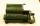May be the sum of two integers less than their difference?
• A number 2A number decreased by the difference between four and the number
• Pounds3 pounds subtract 1/3 of a pound.
• Opposite numbers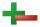Calculate opposite numbers (additive inverse) to given ones:How much and how many times is 72.1 greater than 0.00721?
• Celsius 25 degrees Celsius at midday dropped 12°Celsius degree by evening. What is the temperature?What is 4 1/2+2/7-213/14?
• Evaluate expressionIf x=2, y=-5 and z=3 what is the value of x-2y
• Mixing operations with numbersSubtract twice the number -23.6 from the difference of the numbers -130 and -40.2.
• The temperature 2The temperature of Siberia fell13 degrees below 2/3 degrees centigrad. What is the temperature there now?
• Expression 6Evaluate expression: -6-2(4-8)-9Add and write the result again as hours, minutes, seconds: 2hodiny45min15s + 1h20m50s =
• Minutes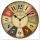Determine the difference in minutes: T1 = 2 3/20 h T2 = 2.3 h
• Mr. TolentinoMr. Tolentino own a piece of land, it measures 86.12 meters long 68.293 meters width. How much longer is the length than the width?
• 8 degrees8 degrees Celsius it cools down by 9 degrees Celsius. What is the resultant temperature?
• Brackets 2Add parenthesis to make true: 5-2×6-4+2=5
• Angles in triangle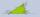Calculate the alpha angle in the triangle if beta is 61 degrees and 98 gamma degrees.
• With bracket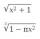Calculate (evaluate) simple mathematical expression with a negative numbers and a bracket: 13+15*5-2*(-6)
• Complementary angles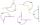Two angles are complementary. If one angle measures 10°, what is the measure of the second angle?

Do you have an interesting mathematical word problem that you can't solve it? Submit a math problem, and we can try to solve it.

We will send a solution to your e-mail address. Solved examples are also published here. Please enter the e-mail correctly and check whether you don't have a full mailbox.

Please do not submit problems from current active competitions such as Mathematical Olympiad, correspondence seminars etc...